# Foundations of geometry

(diff) ← Older revision | Latest revision (diff) | Newer revision → (diff)

A branch of geometry in which the basic concepts of geometry, the relations between them and related questions are studied.

The important role of basic concepts and the relations between them, on which the definitions of figures are based and geometric propositions are proved, was recognized in the works of even the ancient geometers. Thus, by developing the deductive method in geometry, they highlighted the special role of the basic concepts, axioms and postulates which constitute the fundaments of geometry. In Euclid's(3rd century B.C.), a chain of definitions of all concepts used in subsequent expositions preceeds the axioms and postulates. Among these definitions, the concepts "point" , "straight line" and "plane" are of crucial importance; their definitions do not depend on other geometric concepts. The actual definitions of these fundamental concepts from the geometric point of view are unsatisfactory, since they express only characteristic physical properties (for example, "a point is that which does not have parts" , i.e. a point is understood to be a small, physically indivisible body). For this reason, the works of other geometers which appeared at almost the same time as the Elements contain numerous comments and critical analyses of the definitions of these basic and other geometric concepts, axioms and postulates. However, these were merely refinements which did not affect the foundations of the definitions. In essence, the proofs of many geometric theorems were based on the clearness of the drawing and the physical feasibility of the necessary geometric constructions, and were not strictly logically deduced from the axioms and postulates. Only in the 19th century, and particularly in the early 20th century, did works appear which explained the full significance of the basic concepts and the relations between them for a logically faultless deductive method for the construction of geometry and its foundations. This deeper analysis of the foundations of geometry was enhanced by the discovery in 1826 of the non-Euclidean Lobachevskii geometry. Results justified by the use of Euclidean geometry on the basis of the same principles and concepts as in the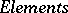appeared in the works of G. Peano (1894), M. Pasch (1882), M. Pieri (1899), D. Hilbert, and others. The Hilbert system of axioms of Euclidean geometry (1899) attracted the most attention. Seeking a logically satisfactory construction of Euclidean geometry, Hilbert distinguished 5 groups of axioms and showed them to be necessary and sufficient for the construction of all of Euclidean geometry. Moreover, he first proposed the logical processing of the whole system, cleared the consistency of the system by the construction of a numerical model and established the independence of the groups of axioms, as well as the completeness of the system. Unlike in the, where the concept of space was described as a "place" for all figures, Hilbert considers a space to be the set of all "points" , "lines" , "planes" , and figures constructed on the basis of these concepts.

The set of basic concepts in Hilbert's system was borrowed (and refined) from the, although this system is in essence a pure geometric scheme, free of references to the clearness of a drawing. Moreover, the language of geometry based on Hilbert's system hardly differs from the language of the.

At almost the same time as Hilbert's system, other systems of axioms of Euclidean geometry appeared. Thus, in F.H. Schur's system (1909), the basic concepts were "point" , "segment" , etc., while instead of the "congruence" of figures in this system, he introduced the concept of a "motion" . This concept made it possible to introduce a group approach in geometry to study motions and to put the research methods in an algebraic form. The geometric schemes above do not fully satisfy the demands of a further generalization of the concept of a space and other concepts, nor are they sufficiently "algebraic" .

New approaches to the foundations of Euclidean geometry demanded the creation of a new "language" , which made it possible to carry out corresponding further generalizations of concepts, algebraization of proofs, classification of objects, etc. One of the most widespread schemes of the foundations of Euclidean geometry, in which the possibilities of generalizations and translation of geometric concepts into the language of algebra are incorporated, is the system of axioms proposed by H. Weyl (1916). One of the transcriptions of the Weyl scheme is given below.-dimensional Euclidean space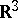is defined as the set consisting of two kinds of elements — "points" and "vectors" — which satisfy the following four groups of axioms:

## Group I.

Axioms which define the relation between points and vectors.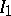. At least one point exists.. To each ordered pair of points corresponds one, and only one, vector.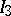. For every pointand every vector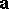, there is one, and only one, pointsuch that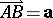(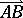is the vector).. If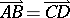, then.

On the basis of this group of axioms the sum of vectors is defined, which satisfies the requirements of commutativity and associativity. There is a zero vector, and every vector has a corresponding negative vector. The vectors with addition form a group.

## Group II.

Axioms which describe the operation of multiplication of a vector by a number.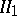. To each vectorand each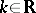corresponds a specific vector(is called the product of the vectorby the number).. Multiplication of a vectorby 1 does not alter the vector.. Multiplication of a vector by a number is distributive with respect to addition of numbers:.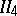. Multiplication of a vector by a number is distributive with respect to addition of vectors:.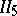. Multiplication of a vector by a number is associative: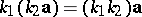.

Using the operations of addition and multiplication by a number, a linear combination of vectors and their linear independence is defined.

## Group III.

The dimension of space is defined.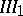. Three linear independent vectors exist, but any four are linearly dependent.

This axiom has a topological character; it follows from this and the second group of axioms thatis a topological space of dimension 3. The first three groups of axioms define a-dimensional affine space.

## Group IV.

The metric properties are defined.. Any two vectorsand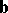correspond to a specific number (the scalar product)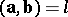,.. Symmetry of the scalar product: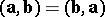.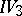. Distributivity of the scalar product: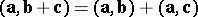.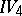. For,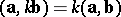.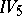. The scalar square of a vector is non-negative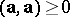, and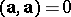only for the zero vector.

On the basis of group IV the distance between points, the angle between vectors, etc., is defined; vectors are used to define "segments" , "straight lines" , "planes" , etc.

The Weyl scheme permits a generalization to the case of arbitrary dimension; using an appropriate change of axioms, this scheme includes hyperbolic and elliptic spaces, etc.

The Weyl system of axioms of Euclidean geometry is consistent, independent, and satisfies the requirement of completeness (categoricity or minimality). Its consistency is established using a numerical model: The "points" of the spaceare in a one-to-one correspondence with ordered sets of numbers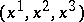,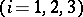. A vector starting at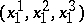and ending at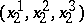is defined by the triple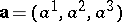,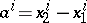. The sum of two vectors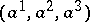andis defined by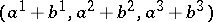, the product of a vectorand a numberis. The scalar product of two vectorsand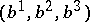is expressed by the number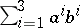. The basis vectors (a triple of linearly independent vectors) can be represented by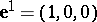,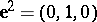,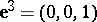. To prove the mutual independence of the axioms and the independence of the groups of axioms, an interpretation of the system is created which is obtained from the given one by replacing any of its axioms by its negation. The completeness of the system is deduced from the completeness of the set of real numbers.

In creating a scheme of Euclidean geometry, a geometric transformation can be taken as basic concept. Thus, in F. Bachmann's system of axioms, symmetry transformations are introduced as a basic concept. Using symmetries, which generate the group of motions in a Euclidean (metric) plane, the "points" and "lines" are defined as involutory elements of this group. Group-theoretic relations are the basis in the definition of the concepts of "incidence" , "orthogonality" , etc., while geometric proofs are replaced by calculations after having been translated into the language of algebra.

The foundations of Euclidean geometry also affect questions relating to the basis of non-Euclidean geometries. In the late 19th century and early 20th century, the basic modern methods and approaches to the foundations of geometry were formulated. New approaches to the concepts forming the foundations of geometry were worked out by B. Riemann, S. Lie, F. Klein, A. Cayley, and others. Among the basic geometric concepts were multi-dimensional manifolds, transformation groups acting on manifolds, invariants of these groups, etc.

The group approach was first accurately formulated in Klein's Erlangen program: A geometric space is defined as a setwith a fixed groupof transformations of it; the study of-invariant properties of the space is the object of geometry. (For example, an-dimensional affine spaceis defined as a set on which the additive group of an-dimensional vector space acts simply transitively.) Lie, Klein and Cayley studied transformation groups, from which arose new possibilities in the classification and foundations of Euclidean and non-Euclidean geometries as geometries of specific transformation groups. Geometry is becoming the study of invariants of transformation groups, and the foundations of geometry rely on group theory.

Riemann worked out the metric approach to the foundations of geometry. A geometric space is considered as a set with a metric which satisfies some axioms. Riemann demonstrated that all internal properties of the space are defined by a given quadratic differential form (curvature, geodesic lines, etc.), which led to the discovery of broad classes of different metric geometries. Initially, spaces and their geometries were classified on a metric basis. Riemann demonstrated the particular role of the choice of coordinates in a point manifold for the study of the quadratic forms themselves. Thus, for a space of constant Riemannian curvature, Riemann introduced a standard form to which a quadratic form can be reduced by means of a corresponding choice of coordinates (cf. Riemannian geometry).

The coordinate method of Euclidean geometry was generalized for various spaces, and was also developed in differential geometry; the concept of a manifold, relying on the choice of coordinate systems, has many uses in geometry. The group approach to research into transformations of differential-geometric objects permitted the creation of the theory of invariants of metric (quadratic) forms. This theory of invariants of transformation groups was the basis for the construction and logical justification of modern differential geometry. The concept of a geometric object became one of the basic concepts, and geometry is considered as the theory of geometric objects (cf. Geometric objects, theory of). The concept of a differentiable manifold makes it possible to give strict definitions of differential-geometric objects and, in particular, to justify methods of analysis in geometry and geometric methods in analysis.

The foundations of Euclidean (and, generally, of any) geometry which depends on a specific system of axioms reveal the special role of set-theoretic principles in the logical analysis of systems of axioms. In fact, the independence and consistency of a system of axioms can be established by constructing a numerical model of this system. Set theory in the foundations of geometry is therefore in its own way a measure of a faultless logical construction of geometric theories. Geometric axioms of continuity (and completeness) are in essence equivalents of set-theoretic axioms.

The construction of a geometry over a specific field is based on the use of concepts of a set-theoretic character. Starting from the creation of Cartesian analytic geometry, the idea of mapping a set of points onto a set of real numbers (or onto an arbitrary number set) has great significance for the foundations of geometry. The development of this idea means that geometries can be defined and classified by means of the number set (usually a field) over which they are constructed.

In the foundations of geometry, set-theoretic methods are widely used in the study of geometric transformations. As observed above, the invariants of transformation groups are the subject of study in the geometry defined by this group. An important use of the theory of invariants of (projective) transformations was discovered by Klein for the interpretation of non-Euclidean geometries. Concepts such as "angle" , "orthogonality" , etc., were analyzed in greater detail. Studies on projective complex spaces and various projective metrizations are of great significance in the classification of spaces with a specific structure.

Topological methods of classification of transformations are also used in the foundations of geometry, and with the aid of these methods the most essential differences between classes and types of manifolds are clarified, and their global properties are studied.

The basic methods and approaches in the foundations of geometry — the synthetic, the group-theoretic and the metric approach — are also significant in modern research in this area of geometry. For example, a generalization of Riemann's ideas on the foundations of geometry is the infinitesimal approach, in which the geometric structure is defined by the specification of a field of certain infinitesimal quantities (e.g. a Finsler metric, a connection, etc.). Many problems of physics and mechanics can be interpreted geometrically and geometric concepts are used in solving them. Generally, all modern systems of axioms of Euclidean (and non-Euclidean) geometry use all three approaches to the foundations of geometry to varying degrees.

The study of tools which can be used in proving theorems on the basis of a given system of axioms is one of the main problems in the foundations of geometry. In the, Euclid used Aristotle's classical logic. Hilbert paid close attention to these questions, outlining the basic problems of mathematical logic. The consistency of systems of geometric axioms is established by constructing numerical models of these systems and by studying them logically.

Questions of measuring segments, areas and volumes are of significance in the foundations of geometry. The concepts of measuring a segment, an area or a volume are based on specific groups of axioms. E.g., the theory of the area of polygons in the Euclidean plane in Hilbert's system of axioms is based on the axioms relating only to the plane and are independent of the axioms of continuity (see Non-Archimedean geometry; Non-Pascalean geometry).

The foundations of geometry deal with the problem of material objective sources of geometric concepts and systems of axioms. One of the principles of constructing geometric systems was, for a long time, the principle of the physical feasibility of a system on some material model. As observed above, an attempt was made already in theto interpret basic concepts from the point of view of their physical properties. In the late 19th century, following the discovery of the Lobachevskii geometry, the question arose once more of the objective possibility of geometries other than the Euclidean. The problem of the objective existence of non-Euclidean geometries was solved by many geometers by using physical models, by which they attempted to prove the independence and consistency of the geometric systems of axioms. Thus, N.I. Lobachevskii attempted to justify the consistency of the conclusions arising from his axiom on parallel lines by using physical measurements of the defects of triangles of gigantic dimensions, the vertices of which are situated on cosmic bodies far from the Earth, in order to prove that the defects are different for different triangles, and that the sum of the interior angles of the triangles can be less than two right angles. An attempt to prove the existence of different metric geometries was made by H. Helmholtz in a work written shortly after the appearance of Riemann's results. Helmholtz gave a physical interpretation to the basic concepts on which metric geometry depends, and laid down certain physical laws regarding the geometric properties of space, which made it possible to construct the geometry of this space. Using a heuristic approach, Helmholtz obtained the metric of space from the basic physical laws as a differential form which, as Riemann demonstrated, defines all the internal properties of the space. Instead of the hypotheses on the foundations of geometry proposed by Riemann, Helmholtz examined facts from which the same conclusions arise in metric geometry, thereby underlining the empirical testing of the validity (consistency) of these conclusions.

Objectively, the works using empirical testing of geometric systems served to spread new geometric ideas and contributed to the appearance of more detailed logical analyses of geometric systems and to the development of the modern basic requirements for these systems. Moreover, attempts at giving geometry physical grounds contributed to the penetration of geometric ideas and methods into different areas of mathematics, physics and mechanics.

The foundations of geometry are of great importance in the methodology of geometry. In teaching modern courses of geometry in universities and pedagogical institutes, the foundations of geometry occupy a central position. In this regard, the choice of a system of basic concepts and axioms plays an every greater role in "shortening" the path between the axioms themselves and the important theorems which can be inferred from them and which have practical uses (especially in solving problems).

How to Cite This Entry:
Foundations of geometry. Encyclopedia of Mathematics. URL: http://encyclopediaofmath.org/index.php?title=Foundations_of_geometry&oldid=13175
This article was adapted from an original article by L.A. Sidorov (originator), which appeared in Encyclopedia of Mathematics - ISBN 1402006098. See original article